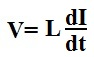﻿ Inductor Equations ﻿# Inductor EquationsBelow is a table of inductor equations.

This table includes formulas to calculate the voltage, current, inductance, impedance, and time constant of an inductor circuit.

Inductor Equations Table
 Equation Description Unit Formula Voltage This equation calculates the voltage that falls across an inductor. Volts(V)Current This equation calculates the current that goes through an inductor. Amperes(A)Inductance This equation calculates the inductance of an inductor. Henry(H)Impedance This equation calculates the impedance of an inductor. Ohms(Ω)Capacitor Time Constant This equation calculates the time constant of an RL circuit. Seconds(S)Now we'll go over the above equations below in more depth, so that you can get a greater undertstanding of these various inductor formulas.

So in calculating the voltage across an inductor, the voltage is equal to the inductance of the inductor times the change in current across the inductor. An inductor is a device that resists change in the flow of current through it. However, as there is change in the current through an inductor, the voltage across the inductor changes. Aas the current flowing through the inductor increases, the voltage across the inductor increases. As the current flowing across the inductor decreases, the voltage across the inductor decreases.

In the next equation, we calculate the current flowing through an inductor. The current across an inductor is equal to the integral of the voltage across the inductor multiplied by the inverse of the inductance plus whatever initial current there was flowing across the inductor. If there was no initial current flowing through the inductor, then I0 is equal to 0.

The next equation calculates the inductance of an air-filled solenoid. This formula calculates the inductance, L, based on the product of the number of turns, N, across the conductor and the linking magnetic flux, Φ, divided by the current, I, producing the flux, according to the formula, L=(NΦ)/I

In the next equation, we calculate the impedance of the inductor. This is the resistance that an inductor offers in a circuit depending on the frequency of the incoming signal. If the signal is a DC signal, meaning it has 0Hz frequency, plugging 0 into the formula, XC= 1/2πfC, we get an impedance of 0. To DC voltage, an inductor has a very low impedance, practically seen as nothing, so DC signals flow through an inductor with easy, almost as if the circuit is a short circuit. However, as we increase the frequency of the signal going through the inductor, the inductor offers greater and greater impedance (resistance). At a certain point, a high enough frequency, it's practically as if the inductor is an open circuit, being that it offers such high impedance. So this formula calculates impedance.

The last equation computes the time constant of an RL circuit, a circuit composed of a single resistor and indctor. The result of the quotient of the resistance divided by the inductance is the time constant in unit seconds. If you take this result and find its inverse by taking 1 and dividing it by this time value, you get the frequency. Both are very valuable for many circuits including circuits where you need a particular time period or frequency.

So these are some of the most frequently seen inductor equations. I can't say for a fact that it's all of them, it probably isn't, but it's definitely a good amount and some of the most popular and widely used formulas for inductors. These equations compute everything from the voltage to the current, inductance, and time constant of inductor circuits.

Related Resources